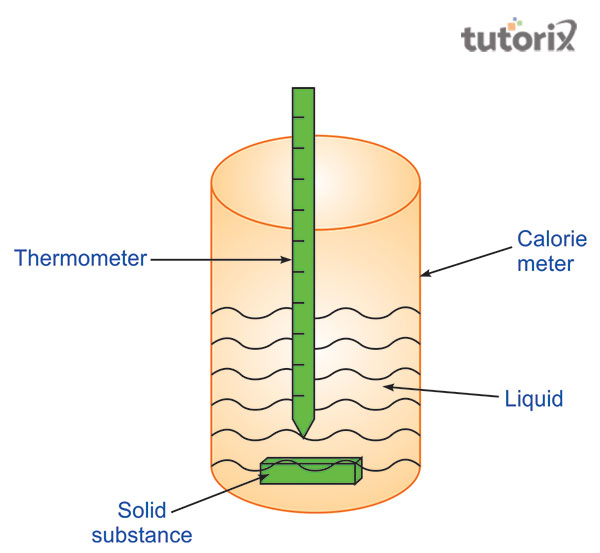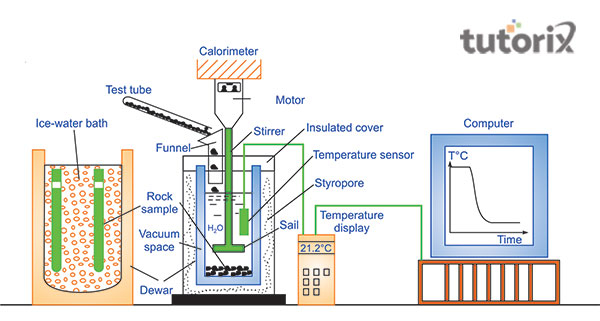# To determine specific heat capacity of given solid by method of mixtures

The quantity of heat required for a substance to reach the temperature of 1oC is usually known by the term specific heat capacity. In order to determine the value of the specific heat capacity of a given solid with the help of a method of mixtures, this specific experiment will be conducted which will be described in this present tutorial. The tutorial will help one to understand the usage of the approach of the mixed for finding a solid’s specific heat capacity.

## Description of the specific heat capacity

The placement of a better-insulated calorimeter will be beneficial for these specific experiments as it will help to get a more accurate result. Experiments conducted previously have observed that the main reason for identifying loss of heat is the conduction which can be generally recognised as an error of the experiment. Therefore, based on such observation, it can be stated that for the current determination of the specific heat test one must need to depend on the conversion of energy (Jamei et al. 2021).

Specific capacity of heat can be determined based on the result of an experienced change in temperature of the water that is considered to be proportional to the heat of the solid

## Apparatus

Prior to initialising the experiment, one must focus on the required materials for the experiment. The required apparatus includes a clamp stand, cold water, two half-degree thermometers, calorimeter and a hypsometer. Another thing that needs to be kept in mind while conducting the experiments will be the required safety precautions in the laboratory along with the arrangements of apparatus mentioned.

## TheoryFigure 1: Determination of specific

The calorimeter is referred to as a device that has been used for the measurement of the flow of heat in a chemical or physical reaction. On the other hand, the hypsometer can be defined as an instrument that is specifically used to determine the point of boiling of liquid like water at a given altitude (Thermtest, 2022). Focusing on this conceptualization, the theory of the experiment evaluates that the loss of heat can be presented as equivalent to the value of measured heat that is gained by the water and calorimeter.

## Procedure

Students who want to measure the specific heat capacity of any solid material are required to follow the mixtures method with the standard procedure to get appropriate results.

• The usefulness of the materials is needed to be determined as the first step in measuring the heat capacity. As the given material is sold as mentioned, it can be referred to as the specific temperature (Ncert, 2022). The following steps are needed to be measured with the process:
• After the determination of the list of required materials and useful theory to measure the heat capacity, the student needs the appropriate determination of the instruments and arranges them properly.
• In the next step, students need to put the two thermometers denoted as A and B inside a beaker including water and note the derivatives. The correction of the point where the thermometer is placed at the point of A is taken as the standard point and the application of the thermometer can make the correct point, as B (Sarbu & Dorca, 2019). Students should place the thermometer in a copper tube in the next stage, which is managing with the inner items contained.
• Based on the available contains, the second or the B thermometer needs to be dipped into the copper tube and the powder that is containing the given powder.
• In the next stage, students need to turn off the burner with a container filled with water (Hlosta et al. 2020). The calorimeter needs to be recorded with the weight over the lid. While adding water to the container the temperature needs to be specified as between 5degree to 8degree C.
• In the next stages, the water needs to be stirred slowly by adding more power in the solid form from the hypsometer to the calorimeter and the final temperature needs to be recorded. In the last step, removing the thermometer will help in getting the final value for this experiment.Figure 2: Methods to measure specific heat capacity

## Precautions

In order to avoid different types of errors, the precautions for this are the followings −

An adequate quantity of solid powder should be operated to conceal the thermometer’s tip. Enough amount of water needs to be put in a hypsometer to avoid temperature fragmentation. The calorimeter needs to take in a polished format to avoid any radiation. The dew point needs to be measured in the water before putting it.

## Conclusion

In order to determine the specific heat of a solid, the present tutorial has included the theory along with describing the specific heat capacity. The procedures of the experiments have been explained in an understandable manner. In order to make the experiment more understandable, the required materials have been included in the explanation section of the experiment based on the aim stated in the introductory section. Moreover, in the apparatus section, it has been stated that for conducting such experiments one must need to have a hypsometer and calorimeter along with the other materials. In addition, a section has been included in this tutorial where the precautions required in this experiment have been listed as well.

## FAQs

Q1. Why is a calorimeter constructed of copper is operated in the tutorial?

The specific amount of heat for copper is low, which seems the liberation and the absorptions of heat will be found in less amount during the transformation of heat.

Q2. What are the sources of errors while measuring the specific heat?

Due to radiation, heat loss can happen. The thermometer’s bulbs might not be fully inside the concrete material causing some errors.

Q3. What is the specific heat of the substance?

The heat capacity of a substance at 1-degree Celsius is often useful for the determination of the mixtures method for the solid item.

Updated on: 13-Oct-2022

681 Views